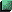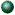# Finite Element Method

## Course Numbers: 20-MECH-7052 or 20-AERO-7052;   Spring Semester

Instructor: Dr. Yijun Liu, Professor of Mechanical EngineeringCourse SyllabusFEM (Spring Semester)Showcase: Finite Element Analysis in ActionsContents of the Lectures (For FEM and Advanced FEM)Chapter 1. Introduction

• Lecture 1.  Introduction to the Finite Element Method; An Example - Spring Elements
• Lecture 2.  Review of the Linear Elasticity - I
• Lecture 3.  Review of the Linear Elasticity - II
• Lecture 4.  Review of the Linear Elasticity - III
• Homework ProblemsChapter 2. FEM for the Plane Truss and Frame

• Lecture 1.  Bar Element and Coordinate Transformation
• Lecture 2.  Examples; Truss Analysis
• Lecture 3.  Beam Element
• Lecture 4.  Examples; Distributed Loads
• Lecture 5.  More Examples; Frame Analysis
• Lecture 6.  Introduction to ANSYS (Computer Lab Session 1)
• Lecture 7.  Review
• Homework ProblemsChapter 3. Foundations of the FEM - Energy Principles

• Lecture 1.  Introduction
• Lecture 2.  The Principle of Stationary Potential Energy - Multi-DOF Systems
• Lecture 3.  The Principle of Stationary Potential Energy - Elastic Bodies
• Lecture 4.  Rayleigh-Ritz Method; Examples
• Lecture 5.  Interpolations and FEM Formulations
• Lecture 6.  Examples
• Lecture 7.  Review
• Homework ProblemsChapter 4. Displacement-Based Elements for Structural Mechanics

• Lecture 1.  General Formulation for Element Stiffness Matrices and Equivalent Load Vectors
• Lecture 2.  Overview of Element Stiffness Matrices and Equivalent Load Vectors
• Lecture 3.  Use of ANSYS/I-DEAS Master Series (Computer Lab Session 2)
• Lecture 4.  Equilibrium, Compatibility, Convergence and the Patch Test
• Homework Problems
• Computer Lab Assignment No. 1Chapter 5. Straight-Sided Triangles and Tetrahedra

• Lecture 1.  Natural Coordinates on Lines, Triangles and Tetrahedra
• Lecture 2.  FE Formulations on Straight-Sided Triangles and Tetrahedra
• Homework Problems
• Computer Lab Assignment No. 2Chapter 6. Isoparametric Formulation

• Lecture 1.  Introduction; 1-D Isoparametric Element
• Lecture 2.  Plane Bilinear Elements;  the Jacobian Matrix
• Lecture 3.  Use of ANSYS (Computer Lab Session 3)
• Lecture 5.  Hexahedral Elements; Plane Triangular Elements; Tetrahedron Elements
• Homework Problems
• Computer Lab Assignment No. 3Chapter 7. Plates and Shells

• Lecture 1.  Introduction to Plate Theories
• Lecture 2.  Plate Elements
• Lecture 3.  Shells and Shell Elements
• Homework Problems
• Computer Lab Assignment No. 4
• Final Exam Project AssignmentChapter 8. Topics in Structural Mechanics

• Lecture 1.  Symmetries in the FE Modeling and Analysis
• Lecture 2.  Superelements
• Lecture 3.  Preview of the Final Project (Computer Lab Session 4)
• Lecture 4.  Fracture Mechanics Analysis
• Lecture 5.  Solutions of FEM Equations; FEA Error Estimates
• Lecture 6.  Presentations of the Final Projects - I
• Lecture 7.  Presentations of the Final Projects - II
• Homework Problems
• Final Exam Project Report DueChapter 9. Vibration Analysis

• Lecture 1.  Structural Dynamics Equations - Single DOF Systems
• Lecture 2.  Structural Dynamics Equations - Multiple DOF Systems
• Lecture 3.  Mass Matrix
• Lecture 4.  Damping Matrix
• Lecture 5.  Free Vibration Analysis - I
• Lecture 6.  Free Vibration Analysis - II
• Lecture 7.  Use of ANSYS (Computer Lab Session 5)
• Computer Lab Assignment No. 1 (Spring Quarter)
• Computer Lab Assignment No. 2Chapter 10. Frequency Response Analysis

• Lecture 1.  Modal Equations
• Lecture 2.  Modal Method for Frequency Response Analysis
• Lecture 3.  Direct Method for Frequency Response Analysis
• Lecture 4.  Use of ANSYS (Computer Lab Session 6)
• Computer Lab Assignment No. 3Chapter 11. Transient Response Analysis

• Lecture 1.  Direct Methods - Explicit Integration
• Lecture 2.  Direct Methods - Implicit Integration
• Lecture 3.  Modal Methods
• Lecture 4.  Advanced Topics in Dynamic Analysis
• Lecture 5.  Use of ANSYS (Computer Lab Session 7)
• Computer Lab Assignment No. 4
• Final Project AssignmentChapter 12. Nonlinear Analysis

• Lecture 1.  Introduction; Solution Methods
• Lecture 2.  Large Deformation, Elastic-Plastic and Contact Stress Analyses
• Final Project Presentation - I
• Final Project Presentation - II
• Final Project Report Due

Contact Info

E-mail:   Yijun.Liu@uc.edu
Tel.:         (513) 556-4607 (Voice),    (513) 556-3390 (Fax)
S-mail:    Mechanical Engineering, University of Cincinnati, P.O. Box 210072, Cincinnati, OH 45221-0072

 ©  1997-2013 Yijun Liu, University of Cincinnati Last updated April 26, 2013 .